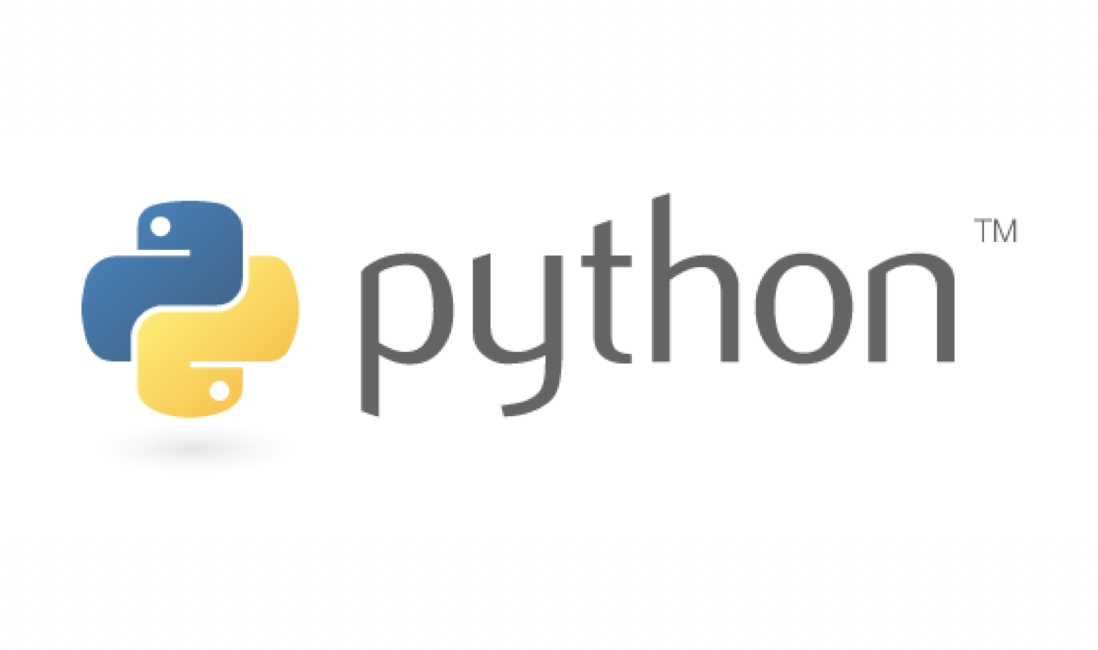### 什么是迭代器，什么是生成器（generator）# 什么是迭代器，什么是生成器（generator）

## 迭代器

``````list = ['a', 'b', 'c']
list_iter = iter(list)  # 创建迭代器
print(next(list_iter))
print(next(list_iter))
print(next(list_iter))``````

``````list = ['a', 'b', 'c']
list_iter = iter(list)  # 创建迭代器
for i in list_iter:
print(i)``````

### 如何创建迭代器

``````import sys

class IncrementNumber(object):
def __iter__(self):
self.num = 1
return self

def __next__(self):
num = self.num
self.num += 1
return num

num = IncrementNumber()
num_iter = iter(num)
for n in num:
if n > 100:
sys.exit()
else:
print(n)``````

`__iter__()`方法返回一个特殊的迭代器对象，这个迭代器对象实现了__next__方法并通过`StopIteration`异常标识迭代的完成

`__next__()`方法会返回下一个迭代器对象

`StopInteration`异常用于标识迭代的完成，防止出现无限循环的情况，

`__next__()`方法中我们可以设置在完成指定循环次数后触发`StopIntertion`异常来结束迭代

``````import sys

class IncrementNumber(object):
def __iter__(self):
self.num = 1
return self

def __next__(self):
if self.num > 50:
raise StopIteration

num = self.num
self.num += 1
return num

num = IncrementNumber()
num_iter = iter(num)
for n in num:
if n > 100:
sys.exit()
else:
print(n)``````

### 生成器

``````import sys

# 生成器函数
def getNum():
yield 1
yield 2
yield 3

# num 是一个迭代器，由生成器返回生成
num = getNum()

while True:
try:
print(next(num))
except StopIteration:
sys.exit()``````